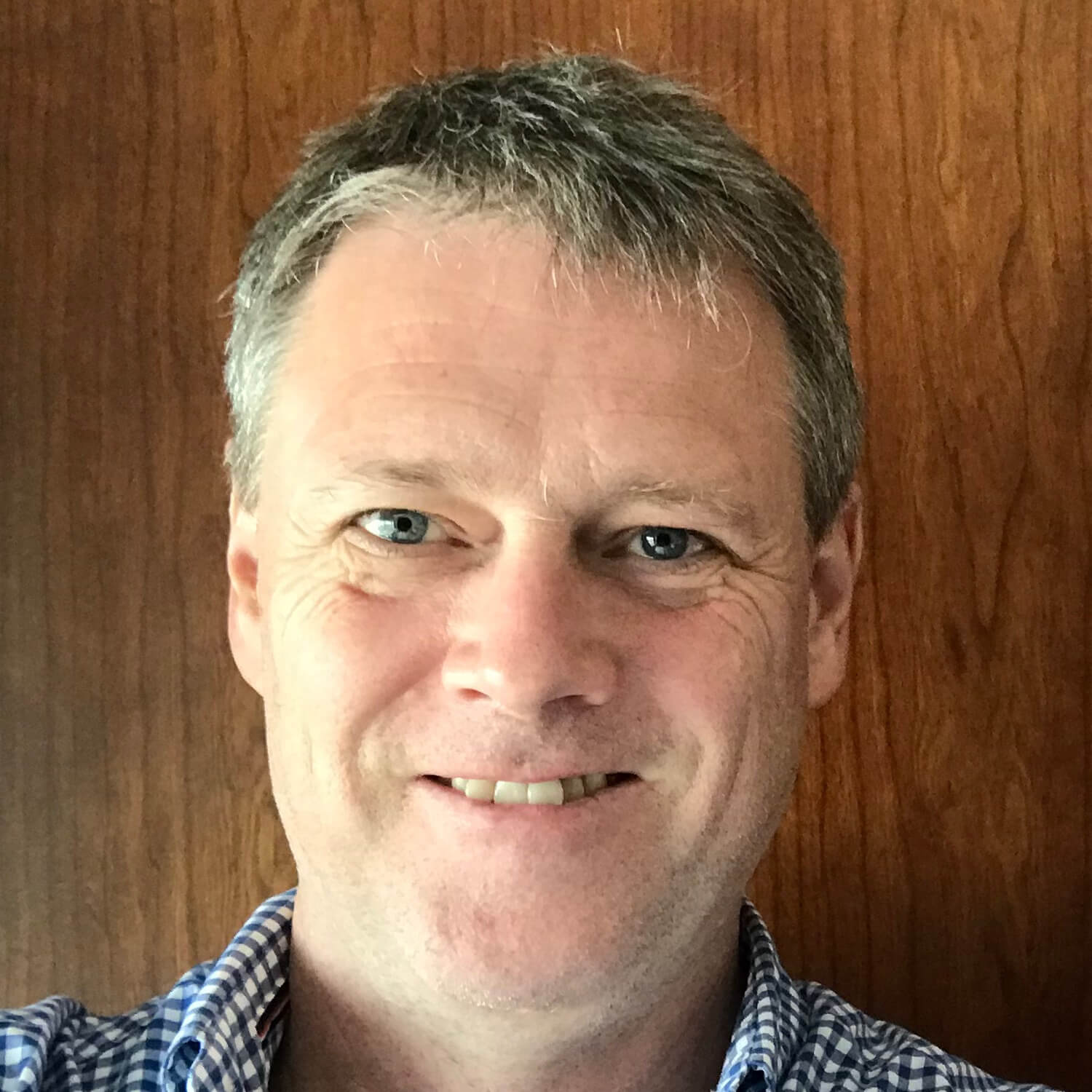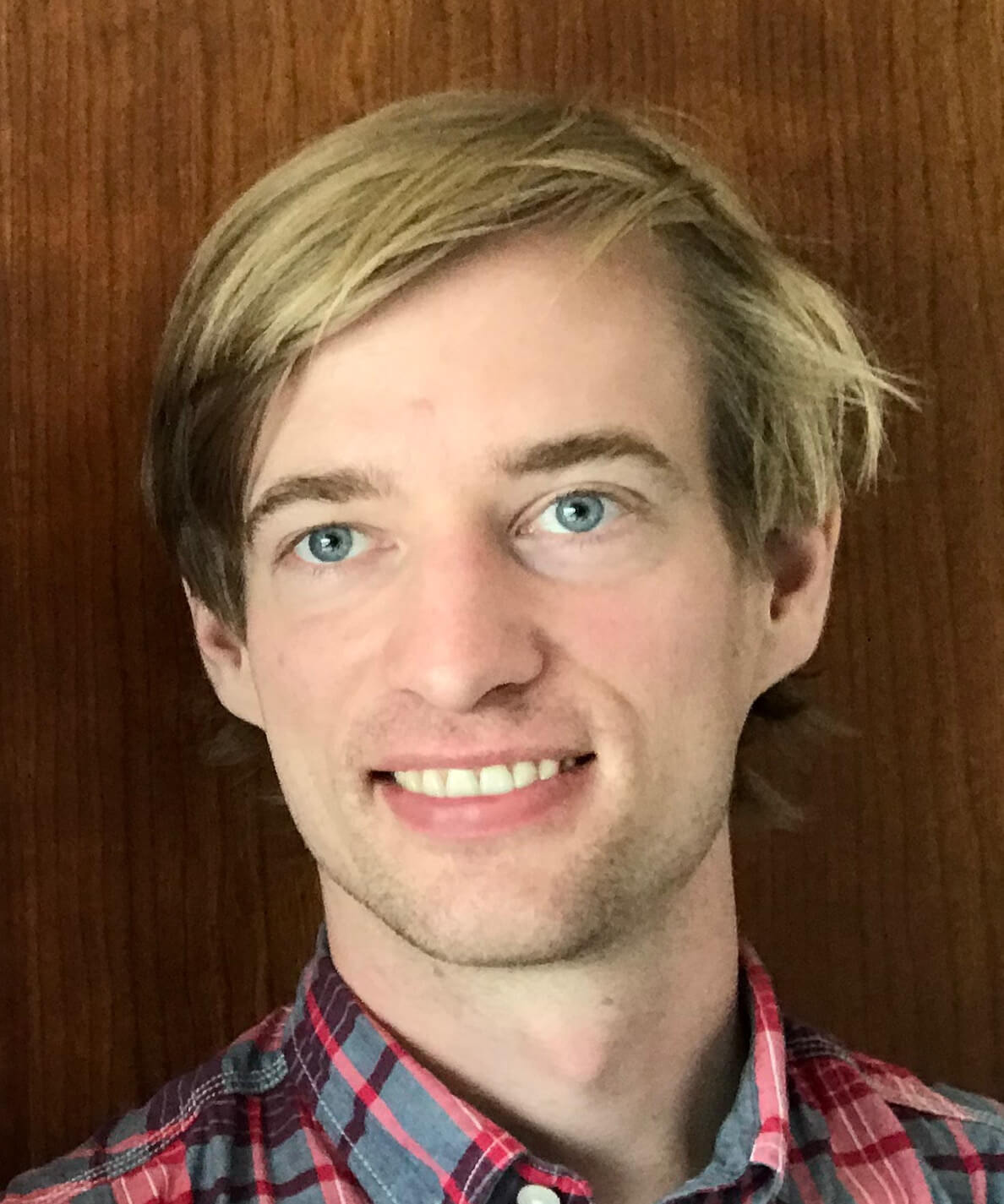#### Lecturers

•Adrian Baddeley
Curtin University – Australia
Spatial Point Patterns: Methodology and Applications with R expand_more

• This course is a practical introduction to statistical methodology for analysing spatial point pattern data. Presentations of the basic principles are followed immediately by hands-on exercises with real data using the R language. Topics include exploratory data analysis, nonparametric estimation of intensity, parametric models (Poisson, Cox, Neyman-Scott, Gibbs), model-fitting and simulation, Monte Carlo tests and model diagnostics. The course gives an in-depth introduction to spatstat, an R package for analysing spatial point patterns. Our recently-published textbook can be consulted for more technical details.

Familiarity with basic statistical concepts, and with the R language for statistical computation, is assumed.Jointly with Ege Rubak (Aalborg University – Denmark).
•Patrick Brown
St. Michael's Hospital and University of Toronto – Canada
Statistical models and inference for spatio-temporal areal data expand_more

• This course will focus on the most common type of areal data, which are counts of incident cases of a health outcome in areas or regions over time. The focus will be on practical aspects of using these models, as well as interpretation and presentation of results. Computer code using R and INLA will be explained and examples will be provided for participants to run. Markov random field (or conditional autoregressive) models, where risk or intensity in each region depends on risk in neighbouring regions, are the basis of the standard and methods and tools for area-level problems. Various forms of spatial and spatio-temporal Markov random field models will be presented, and Bayesian inference for these models will be motivated and described. The course will conclude with the problem of changes in the spatial boundaries over time (i.e. changes in census regions), and methodology and software using the local-EM algorithm will be explained. add
•Håvard Rue
KAUST – Saudi Arabia
Spatial and spatio-temporal models using the SPDE-approach expand_more

• In these lectures we will discuss how to construct (Gaussian) spatial models, in space and space-time, using stochastic partial differential equations (the so-called SPDE approach), and how to do Bayesian analysis of these models efficiently using R-INLA. The SPDE-approach comes with several benefites. First, it generates models, like the Gaussian fields with the Matern covariance function, that is extremely well suited for practical computations through sparse precision matrices. Secondly, it is the natural framework for constructing non-stationary models, like accounting for barriers, and non-separable models in space-time. addJointly with Haakon Bakka (KAUST – Saudi Arabia).
•Liliane Bel
AgroParisTech – France
New trends in spatio-temporal geostatistics expand_more

• Spatial and spatio-temporal prediction techniques need the modelling of covariance function. We will investigate in this course methods for modelling and estimating the covariance function in some special frameworks : no separable spatio-temporal covariance, covariance on the sphere, estimating the covariance function with massive datasets, non stationary processes.
Examples on real datasets will be studied, and R packages devoted to covariance estimation, spatio-temporal prediction and simulation will be presented.